## martes, 30 de enero de 2018

### Selecting evolutionary models in phylogeny

Introduction

Both Maximum Likelihood (ML) and Bayesian Inference (IB) use evolutionary models, which are used to predict substitution rates in molecular sequences (through probabilities) along the branches of a phylogenetic tree. That is, a substitution model describes the process by means of which a sequence of characters is transformed into another set of homologous states over time (Lio and Goldman, 1998). ML and IB are based on the Likelihood function, which needs explicit models of evolution to capture the underlying evolutionary processes of the sequence data (Lou et al, 2010). The majority of models taken into account in these two methodological approaches consist of modifications of the GTR model, in which both the nucleotide change and the frequency thereof can take different values (Huelsenbeck et al, 2004). Given that the evolutionary model chosen for the ML and IB analyzes can exert significant influence on the obtained phylogenetic tree (Lou et al, 2010); I propose to evaluate the sensitivity of THE? phylogenetic reconstruction to the choice of the evolutionary model under the ML method, as well as the effectiveness of the information and hLRT criteria.

Methodology

Initially, a topology for a ten-terminal ultrametric tree was generated randomly, from which ten nucleotide sequences were simulated, with a total length of 1000 bp according to each parameter to be taken into account, generating three Groups "Models", "Models + G "," Models + I "and" Models + G + I ", each and every one of them in the SeqGen software v1.3.4.

For each group, the models to be taken into account were JC, K2P, F81, HKY, GTR. After having the nucleotide sequences the trees were reconstructed under the ML method, with ten replications in PhyML v3.1. The comparison of "Models" with respect to the rest of the groups was made through the Robinson-Foulds metric or symmetric difference.

Regarding the evaluation of the information criteria (BIC and AIC) and hLRT, the evolutionary model was calculated in JmodelTest v2.1.10, reporting reporting the number of models found that corresponded to the initial models with which the sequences were calculated, as well as the frequency in which each model and group was recovered according to the criteria.

Results and discussion

The evaluation of the models by group reports that the group "Model" and "Model_G" are the ones that present lower values of RF with respect to all the evolutionary models evaluated, these being, therefore, the most similar to the reference topology indicating that if At the moment of choosing the evolutionary model for a phylogenetic reconstruction, parameters such as I or I + G could be considered, adding noise, generating not so true topologies (Fig. 1). Likewise, the models, in general, show a similar behavior with respect to the RF values (Fig. 1), however, it is worth highlighting the particular model of JC that under the groups "Models" and "Models_G" are the most similar to the reference topology, perhaps because of the simplicity of it. However, it is important to keep in mind that these particular models depend very much on the heterogeneity given by the nucleotide sequence.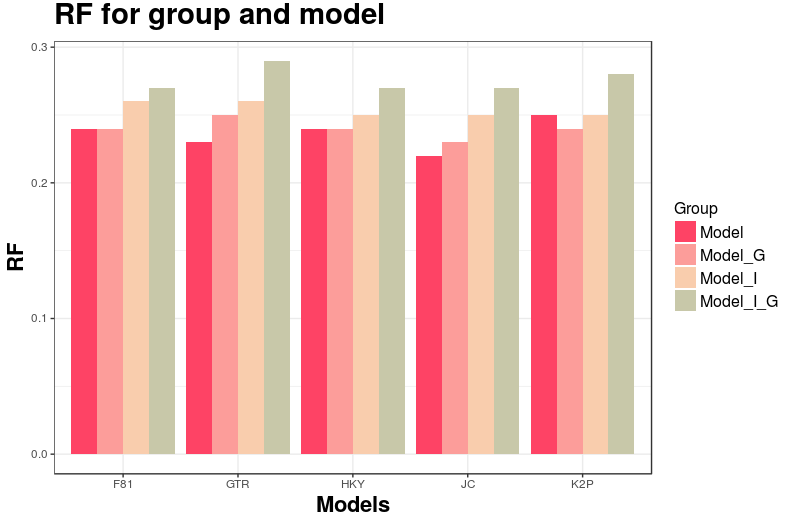Fig 1. RF values for each model, according to the group given the reference topology.

The F81 model presented higher frequency with respect to the BIC criterion and, together with the GTR, it presented the highest frequency with respect to the AIC and hLRT criteria. In general, the frequency values for the different criteria were relatively similar, highlighting some differences in criteria such as BIC with F81 and AIC with HKY (Fig. 2). Likewise, F81 is the model reported by the criteria that coincides to a greater extent with respect to the initial models given by the calculated sequence (Fig 3.). With the exception of some particular cases, it could be said that in general the three criteria evaluated - or at least two of them in some cases - will always tend to choose the same model, being rare the case in which the three criteria choose models completely different, which agrees with that reported by Luo et al (2010) and Ripplinger et al, (2008).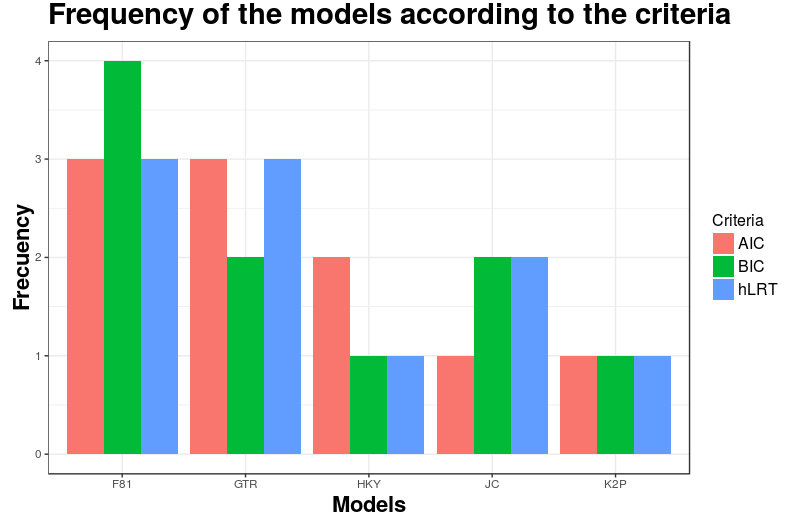Fig 2. Frequency of the models found by each criterion.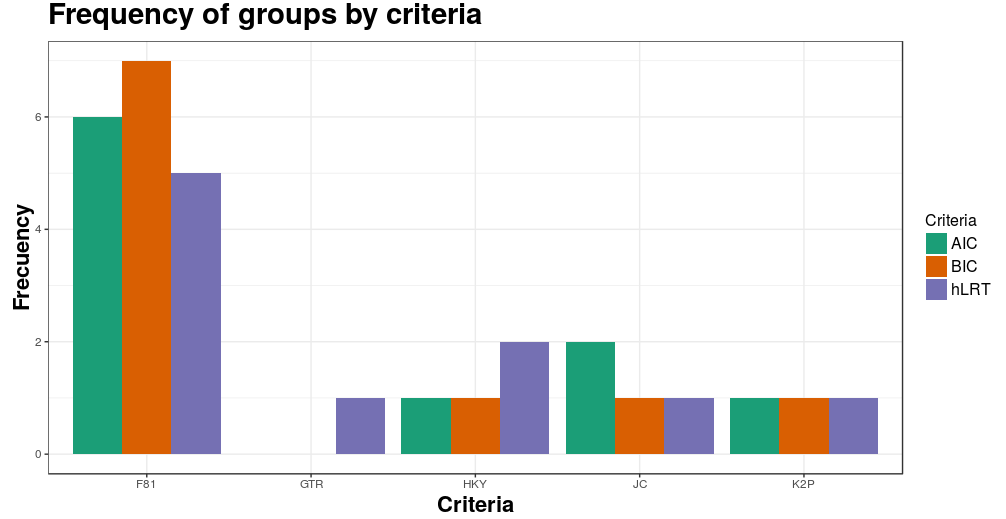Fig 3. Frequency of the models by criteria given the models initially proposed by the nucleotide sequences.

Criteria such as AIC and BIC tend to choose between models with less variation, likewise hLRT tends to choose models with more parameters such as G, I and I + G (Fig. 4). It should also be noted that among the models with more parameters, AIC and BIC always tend to choose those with the lowest possible variation, such as Model + G or Model + I, with Models + G + I being the one with the lowest frequency. The three criteria find the group "Models" as the one that most matches the initial models proposed with the sequences (Fig. 5).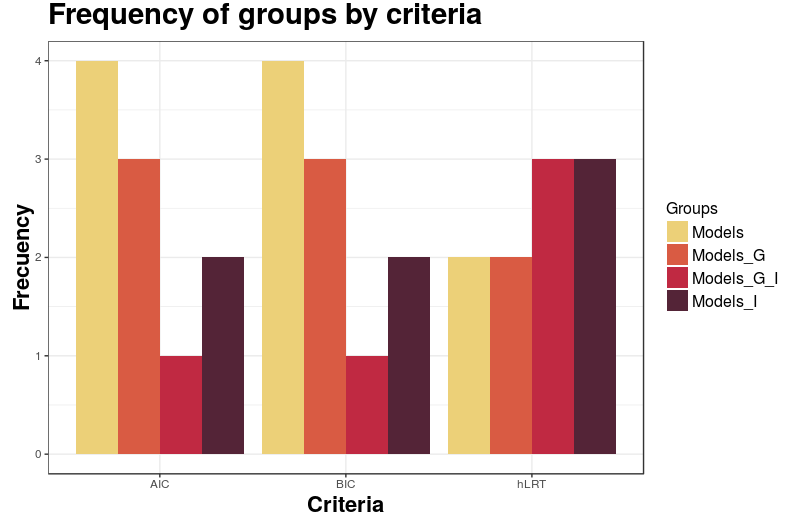Fig 4. Frequency of the groups given all the criteria used.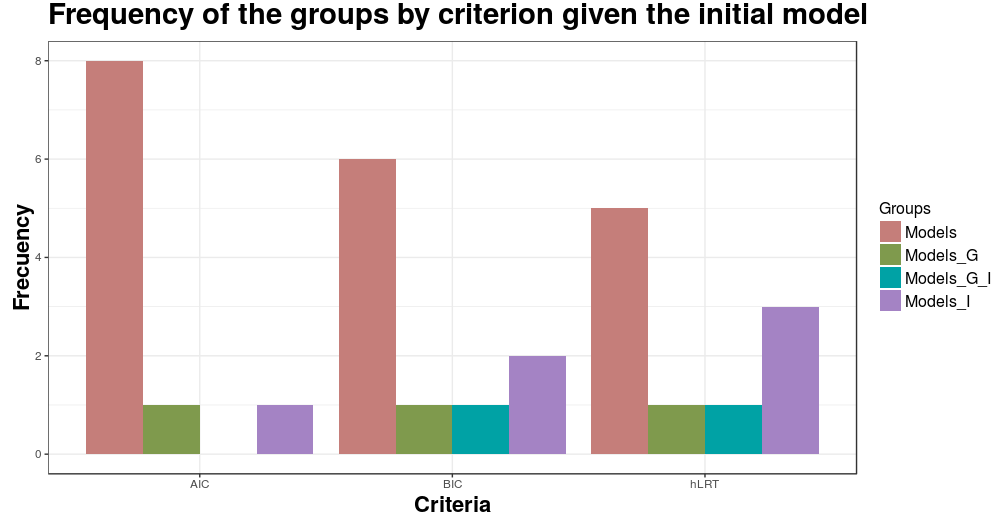Fig 5. Groups with higher frequency by criteria given the groups initially proposed by the nucleotide sequences.

References

-Luo et al (2010). Performance of criteria for selecting evolutionary models in phylogenetics: a comprehensive study based on simulated datasets. BMC Evolutionary Biology. 10: 242.
- Ripplinger J, Sullivan J (2008). Does choice in model selection affect maximum likelihood analysis? Syst Biol, 57 :76-85.
- Posada D, Crandall KA (2001) Selecting the best-fit model of nucleotide substitution. Syst Biol, 50 :580-601.
- Posada D (2008). jModelTest phylogenetic model averaging. Mol Biol Evol. 25 :1253-1256.
- Rambaut A, Grassly NC (1997). Seq-Gen: an application for the Monte Carlo simulation of DNA sequence evolution along phylogenetic trees. Comput Appl Biosci, 13 :235-238.
- Guindon S. et al. (2010). New Algorithms and Methods to Estimate Maximum-Likelihood Phylogenies: Assessing the Performance of PhyML 3.0. Systematic Biology, 59(3):307-21.